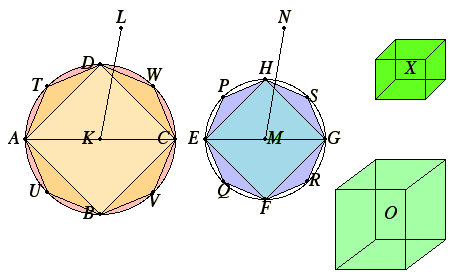# Proposition 11

Cones and cylinders of the same height are to one another as their bases.

Let there be cones and cylinders of the same height, let the circles ABCD and EFGH be their bases, KL and MN their axes, and AC and EG the diameters of their bases.

I say that the circle ABCD is to the circle EFGH as the cone AL is to the cone EN.For, if not, then the circle ABCD is to the circle EFGH as the cone AL is either to some solid less than the cone EN or to a greater.

First, let it be in that ratio to a less solid O, and let the solid X be equal to that by which the solid O is less than the cone EN. Therefore the cone EN equals the sum of the solids O and X.

IV.6

Inscribe the square EFGH in the circle EFGH. Therefore the square is greater than the half of the circle.

XII.6

Set up from the square EFGH a pyramid of equal height with the cone. Therefore the pyramid so set up is greater than the half of the cone, for if we circumscribe a square about the circle, and set up from it a pyramid of equal height with the cone, then the inscribed pyramid is half of the circumscribed pyramid, for they are to one another as their bases, while the cone is less than the circumscribed pyramid.

Bisect the circumferences EF, FG, GH, and HE at the points P, Q, R, and S, and join HP, PE, EQ, QF, FR, RG, GS, and SH.

Therefore each of the triangles HPE, EQF, FRG, and GSH is greater than the half of that segment of the circle about it.

Set up on each of the triangles HPE, EQF, FRG, and GSH a pyramid of equal height with the cone. Therefore each of the pyramids so set up is also greater than the half of that segment of the cone about it.

X.1

Thus, bisecting the circumferences which are left, joining straight lines, setting up on each of the triangles pyramids of equal height with the cone, and doing this repeatedly, we shall leave some segments of the cone which are less than the solid X.

Let such be left, and let them be the segments on HP, PE, EQ, QF, FR, RG, GS, and SH. Therefore the remainder, the pyramid with the polygonal base HPEQFRGS and the same height as that of the cone, is greater than the solid O.

Now inscribe in the circle ABCD the polygon DTAUBVCW similar and similarly situated to the polygon HPEQFRGS, and on it set up a pyramid of equal height with the cone AL.

Since then the square on AC is to the square on EG as the polygon DTAUBVCW is to the polygon HPEQFRGS, while the square on AC is to the square on EG as the circle ABCD is to the circle EFGH, therefore the circle ABCD is to the circle EFGH as the polygon DTAUBVCW is to the polygon HPEQFRGS.

XII.6

But the circle ABCD is to the circle EFGH as the cone AL is to the solid 0, and the polygon DTAUBVCW is to the polygon HPEQFRGS as the pyramid with the polygonal base DTAUBVCW and the vertex L is to the pyramid with the polygonal base HPEQFRGS and the vertex N.

Therefore the cone AL is to the solid O as the pyramid with the polygonal base DTAUBVCW and vertex L is to the pyramid with the polygonal base HPEQFRGS and vertex N. Therefore, alternately the cone AL is to the pyramid in it as the solid O is to the pyramid in the cone EN.

But the cone AL is greater than the pyramid in it, therefore the solid O is also greater than the pyramid in the cone EN.

But it is also less, which is absurd.

Therefore the cone AL is not to any solid less than the cone EN as the circle ABCD is to the circle EFGH.

Similarly we can prove that neither is the cone EN to any solid less than the cone AL as the circle EFGH is to the circle ABCD.

I say next that neither is the cone AL to any solid greater than the cone EN as the circle ABCD is to the circle EFGH.

For, if possible, let it be in that ratio to a greater solid O. Therefore, inversely the circle EFGH is to the circle ABCD as the solid O is to the cone AL.

But the solid O is to the cone AL as the cone EN to some solid less than the cone AL, therefore the circle EFGH is to the circle ABCD as the cone EN is to some solid less than the cone AL, which was proved impossible.

Therefore the cone AL is not to any solid greater than the cone EN as the circle ABCD is to the circle EFGH.

But it was proved that neither is it in this ratio to a less solid, therefore the circle ABCD is to the circle EFGH as the cone AL is to the cone EN.

XII.10

But the cone is to the cone as the cylinder is to the cylinder, for each is triple each. Therefore the circle ABCD is to the circle EFGH as are the cylinders on them of equal height.

Therefore, cones and cylinders of the same height are to one another as their bases.

Q.E.D.

## Guide

#### Use of this proposition

This proposition is used in the proofs of XII.13 and XII.14 when the cylinders under question have the same height and equal bases, and in the proof of XII.15 for cylinders of different heights.